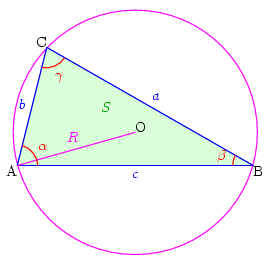# EU

Geometry Level 2Find the minimum value of the expression $\frac{R}{r}$ ,where $R$ and $r$ are the circumradius and inradius of any triangle.

×